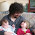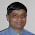## Monday, March 9, 2009

### Calculating probabilities with N throws of a die

A translation of this post in German can be found here.

Chen Shapira pointed me to a SQL riddle by Iggy Fernandez. Laurent Schneider already gave an impressively simple solution using a hierarchical query and the XMLQuery function. I used the SQL model clause (of course) to achieve the same:

`rwijk@ORA11GR1> select * from die order by face_id  2  /   FACE_ID FACE_VALUE PROBABILITY---------- ---------- -----------         1          1         .25         2          3         .25         3          4         .25         4          5  .083333333         5          6  .083333333         6          8  .0833333336 rows selected.rwijk@ORA11GR1> var N numberrwijk@ORA11GR1> exec :N := 2PL/SQL procedure successfully completed.rwijk@ORA11GR1> with number_of_dies as (select count(*) cnt from die)  2  , all_probabilities as  3  ( select sum_value  4         , prob  5         , i  6      from (select level l from number_of_dies connect by level <= power(cnt,:N))  7         , number_of_dies  8     model  9           reference r on (select face_id, face_value, probability from die) 10             dimension by (face_id) 11             measures (face_value,probability) 12           main m 13             partition by (l rn, cnt) 14             dimension by (0 i) 15             measures (0 die_face_id, 0 sum_value, 1 prob, l remainder) 16           rules iterate (1000) until (iteration_number + 1 = :N) 17           ( die_face_id = 1 + mod(remainder-1,cv(cnt)) 18           , remainder   = ceil((remainder - die_face_id + 1) / cv(cnt)) 19           , sum_value   = sum_value + face_value[die_face_id] 20           , prob        = prob * probability[die_face_id] 21           ) 22  ) 23  select sum_value "Sum" 24       , sum(prob) "Probability" 25    from all_probabilities 26   group by rollup(sum_value) 27   order by sum_value 28  /       Sum Probability---------- -----------         2       .0625         4        .125         5        .125         6  .104166667         7  .166666667         8  .104166667         9        .125        10  .048611111        11  .055555556        12  .048611111        13  .013888889        14  .013888889        16  .006944444                     114 rows selected.rwijk@ORA11GR1> exec :N := 3PL/SQL procedure successfully completed.rwijk@ORA11GR1> with number_of_dies as (select count(*) cnt from die)  2  , all_probabilities as  3  ( select sum_value  4         , prob  5         , i  6      from (select level l from number_of_dies connect by level <= power(cnt,:N))  7         , number_of_dies  8     model  9           reference r on (select face_id, face_value, probability from die) 10             dimension by (face_id) 11             measures (face_value,probability) 12           main m 13             partition by (l rn, cnt) 14             dimension by (0 i) 15             measures (0 die_face_id, 0 sum_value, 1 prob, l remainder) 16           rules iterate (1000) until (iteration_number + 1 = :N) 17           ( die_face_id = 1 + mod(remainder-1,cv(cnt)) 18           , remainder   = ceil((remainder - die_face_id + 1) / cv(cnt)) 19           , sum_value   = sum_value + face_value[die_face_id] 20           , prob        = prob * probability[die_face_id] 21           ) 22  ) 23  select sum_value "Sum" 24       , sum(prob) "Probability" 25    from all_probabilities 26   group by rollup(sum_value) 27   order by sum_value 28  /       Sum Probability---------- -----------         3     .015625         5     .046875         6     .046875         7       .0625         8     .109375         9      .09375        10        .125        11  .098958333        12  .104166667        13  .088541667        14  .057291667        15   .05787037        16  .032986111        17  .027777778        18  .012731481        19  .008680556        20  .006944444        21  .001736111        22  .001736111        24  .000578704                     121 rows selected.`

And with a traditional die:

`rwijk@ORA11GR1> update die  2     set face_value = face_id  3       , probability = 1/6  4  /6 rows updated.rwijk@ORA11GR1> select * from die order by face_id  2  /   FACE_ID FACE_VALUE PROBABILITY---------- ---------- -----------         1          1  .166666667         2          2  .166666667         3          3  .166666667         4          4  .166666667         5          5  .166666667         6          6  .1666666676 rows selected.rwijk@ORA11GR1> with number_of_dies as (select count(*) cnt from die)  2  , all_probabilities as  3  ( select sum_value  4         , prob  5         , i  6      from (select level l from number_of_dies connect by level <= power(cnt,:N))  7         , number_of_dies  8     model  9           reference r on (select face_id, face_value, probability from die) 10             dimension by (face_id) 11             measures (face_value,probability) 12           main m 13             partition by (l rn, cnt) 14             dimension by (0 i) 15             measures (0 die_face_id, 0 sum_value, 1 prob, l remainder) 16           rules iterate (1000) until (iteration_number + 1 = :N) 17           ( die_face_id = 1 + mod(remainder-1,cv(cnt)) 18           , remainder   = ceil((remainder - die_face_id + 1) / cv(cnt)) 19           , sum_value   = sum_value + face_value[die_face_id] 20           , prob        = prob * probability[die_face_id] 21           ) 22  ) 23  select sum_value "Sum" 24       , sum(prob) "Probability" 25    from all_probabilities 26   group by rollup(sum_value) 27   order by sum_value 28  /       Sum Probability---------- -----------         3   .00462963         4  .013888889         5  .027777778         6  .046296296         7  .069444444         8  .097222222         9  .115740741        10        .125        11        .125        12  .115740741        13  .097222222        14  .069444444        15  .046296296        16  .027777778        17  .013888889        18   .00462963                     117 rows selected.rwijk@ORA11GR1> exec :N := 4PL/SQL procedure successfully completed.rwijk@ORA11GR1> with number_of_dies as (select count(*) cnt from die)  2  , all_probabilities as  3  ( select sum_value  4         , prob  5         , i  6      from (select level l from number_of_dies connect by level <= power(cnt,:N))  7         , number_of_dies  8     model  9           reference r on (select face_id, face_value, probability from die) 10             dimension by (face_id) 11             measures (face_value,probability) 12           main m 13             partition by (l rn, cnt) 14             dimension by (0 i) 15             measures (0 die_face_id, 0 sum_value, 1 prob, l remainder) 16           rules iterate (1000) until (iteration_number + 1 = :N) 17           ( die_face_id = 1 + mod(remainder-1,cv(cnt)) 18           , remainder   = ceil((remainder - die_face_id + 1) / cv(cnt)) 19           , sum_value   = sum_value + face_value[die_face_id] 20           , prob        = prob * probability[die_face_id] 21           ) 22  ) 23  select sum_value "Sum" 24       , sum(prob) "Probability" 25    from all_probabilities 26   group by rollup(sum_value) 27   order by sum_value 28  /       Sum Probability---------- -----------         4  .000771605         5   .00308642         6  .007716049         7  .015432099         8  .027006173         9  .043209877        10  .061728395        11  .080246914        12  .096450617        13  .108024691        14  .112654321        15  .108024691        16  .096450617        17  .080246914        18  .061728395        19  .043209877        20  .027006173        21  .015432099        22  .007716049        23   .00308642        24  .000771605                     122 rows selected.`

About the solution: it generates as much rows as there are combinations (power(cnt,:N)). With the die_face_id and remainder measures, each generated row is converted to N face_id's in N iterations. The face_value and probability numbers are looked up using a reference model. Finally, the outer query just sums up all probabilities.

The SQL language is "not complete", but since version 10 I seriously wonder if there are problems out there that cannot be solved using SQL. Although it may look like a pretzel to some, with some comments I'm sure it's not that hard to maintain.

#### 8 comments:

1.Rob,

What can I say? Another post from you and a week's worth of homework for me to understand the "solution" and more importantly, the thought process (or the "How" factor)...:)

p.s. I hope you are not serious about your last statement with some comments I'm sure it's not that hard to maintain....:)

2.Hi Narendra,

I was serious with that statement. When you think about the problem and what needs to be done to calculate the probabilities, I definitely think this model clause query is not that hard. Especially when compared to alternatives.

I will address this comment in a future blog post in a more generic way.

Regards,
Rob.

3.hi Rob (and especially Narenda)

It's well worth a compare and contrast with Alberto Dell'era 's mathematical approach at http://www.adellera.it/investigations/nocoug_challenge/index.html . I'm reasonably certain that the model clause approach will be broadly understandable and more simply maintained (if somewhat slower :( ).

I'm very grateful though to both Rob and Alberto for demonstrating both the power of sql and the mindset of an effective developer.

4.Hi Niall,

Thanks for the comment and the link. And wow, what a great solution by Alberto!

Regards,
Rob.

5.The Second International NoCOUG SQL Challenge has been published in the February 2011 issue of the NoCOUG Journal. The Journal can be downloaded from http://bit.ly/gVNZsW. SQL commands for creating the required data are available at http://bit.ly/g58WVn.

6.Hi Iggy,

Thanks for the links. I had already seen the journal and the 2nd SQL Challenge. Unfortunately, I don't understand what needs to be done. Is it forming a sentence? Three sentences? Do all words need to be used? If so, lots of sentences can be made; how do I know which is the right one? I'm afraid I don't think it is a *SQL* Challenge, but I may be missing something.

Regards,
Rob.

7.Hi Iggy,

As already expressed in my email, I now understand that what initially looks like missing information about the challenge, is actually part of the fun.

Thanks for organizing the second challenge. It definitely was a challenge.

Regards,
Rob.

8.Hello Rob,

I was going through this blogs calculating-probabilities-with-n-throws and i was debugging how model clause here is generating various combinations like suppose if it is a 2 face die and rolled 3 times, so these will be face_id combinations

[1,1,1]
[1,1,2]
[1,2,1]
[1,2,2]
[2,2,1]
[2,2,2]
[2,1,1]
[2,1,2]

face_id face_value probability
1 1 0.25
2 2 0.25

How the rules are exactly evaluating to generate various combinations ?

measures (0 die_face_id, 0 sum_value, 1 prob, l remainder)

is remainder a incremental variable for each row processed by model
clause?
First 2^3 ( 2 face id , 3 times rolled) rows are generated and then
rules are applied over each row to calcualte sum and prob .

can you please explain it a more ? this will help understand working of model clause